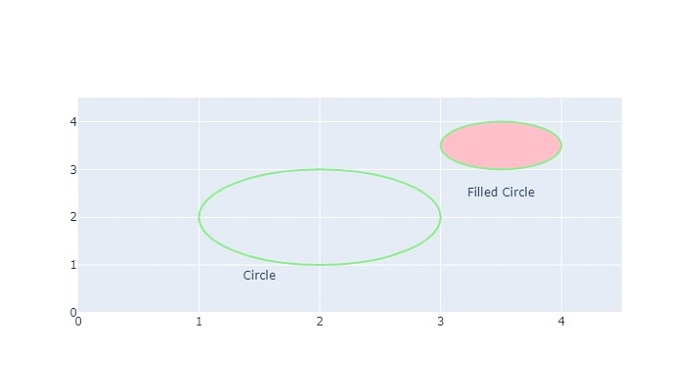# Python Plotly ŌĆō How to simultaneously apply color/shape/size in a Scatter Plot?

Plotly is an open-source Python library for creating interactive web-based charts. In this tutorial, we will show how you can Plotly to apply color/shape/size in a Scatter Plot.

• Here, we will use the plotly.graph_objects module to generate figures. It contains a lot of method to generate charts.

• In addition, we will use the add_trace() method to create a scatter plot and then use the add_shape() method to set the type, shape, and line color.

• Finally, we will use the update_layout() method to set the height and width of the chart.

Follow the steps given below to apply color/shape in a scatter plot.

Step 1

Import the plotly.graphs_objs module and alias as go.

import plotly.graphs_objs as go


Step 2

Use the add_trace() method to generate the scatter plot.

# Create scatter trace of text labels
x=[1.5, 3.5],
y=[0.75, 2.5],
text=["Circle", "Filled Circle"],
mode="text",
))


Step 3

Use the update_axes() method to update the traces of the Scatter plot.

# Set axes properties
fig.update_xaxes(range=[0, 4.5], zeroline=False)
fig.update_yaxes(range=[0, 4.5])


Step 4

Use the add_shape() method to add two circle shapes on the chart.

# Add circles
fig.add_shape(type="circle", xref="x", yref="y", x0=1, y0=1, x1=3, y1=3, line_color="LightGreen", )
fig.add_shape(type="circle", xref="x", yref="y", fillcolor="pink", x0=3, y0=3, x1=4, y1=4, line_color="LightGreen",)


Step 5

Use the update_layout() method to set the height and width of the chart ŌłÆ

# Set figure size
fig.update_layout(width=800, height=800)


## Example

The complete code to apply color/shape/size is as follows ŌłÆ

import plotly.graph_objects as go
fig = go.Figure()
# Create scatter trace of text labels
x=[1.5, 3.5],
y=[0.75, 2.5],
text=["Circle", "Filled Circle"],
mode="text",
))

# Set axes properties
fig.update_xaxes(range=[0, 4.5], zeroline=False)
fig.update_yaxes(range=[0, 4.5])

fig.add_shape(type="circle", xref="x", yref="y", x0=1, y0=1, x1=3, y1=3, line_color="LightGreen",)

fillcolor="pink", x0=3, y0=3, x1=4, y1=4, line_color="LightGreen", )

# Set figure size
fig.update_layout(width=716, height=400)
fig.show()


## Output

On execution, it will show the following output on the browser ŌłÆSimilarly, you can try creating different types of graphs as images.